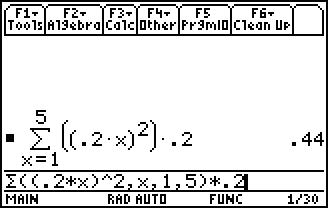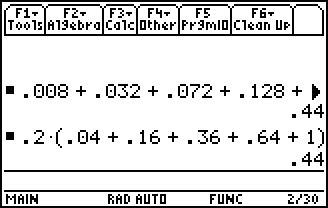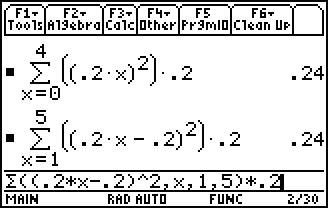# Activities

••• ##### Subject Area

• Math: Calculus: Antiderivatives and Slope Fields
• Math: Calculus: Limits of Functions

• ##### Author9-12

45 Minutes

• ##### Device
• TI-89 / TI-89 Titanium

## Exploring the Area Under a Curve#### Activity Overview

Students explore Riemann sums to find the approximate area under the graph of a parabola on the interval [0, 1].

#### Key Steps

•Students focus on two questions that define the activity:

How can you use rectangles to approximate the area under the curve y = x2 and above the x-axis?

Is there a way to use rectangles to find the exact area under the curve?

Students discuss the goal of the activity, which is to use rectangles to approximate the area under a curve.

•Students make use of five right-endpoint rectangles to find an approximation of the area under the curve.

Students will be introduced to the Riemann sum. They explore the Riemann sum with right-endpoints.

•Next, students use five left-endpoint rectangles to find an approximation of the area under the curve.

Students will see that the first rectangle has a height of zero and unlike the right-endpoint approximation a unit of measure shifts down each value of x.